Search
•Nagesh Singh Chauhan

# Fourier Transformation for a Data Scientist

Updated: May 15

The article contains a brief introduction to Fourier transformation mathematically and its applications in AI.Credits: Shutterstock

# Introduction

The Fourier Transform is one of the deepest insights ever made in mathematics but unfortunately, the meaning is buried deep inside some ridiculous equations.

It is a way of splitting something up into a bunch of sine waves. As usual, the name comes from a person who lived a long time ago called Fourier.

In mathematical terms, The Fourier Transform is a technique that transforms a signal into its constituent components and frequencies.

Fourier transform is widely used not only in signal (radio, acoustic, etc.) processing but also in image analysis eg. edge detection, image filtering, image reconstruction, and image compression. One example: Fourier transformation of transmission electron microscopy images helps to check the periodicity of the samples. periodicity — means pattern. Fourier transform of your data can expand accessible information about the analyzed sample.

To understand it better consider a signal x(t):If we do the same for another signal and select the same moment in time and measure its amplitude.

Consider another signal y(t):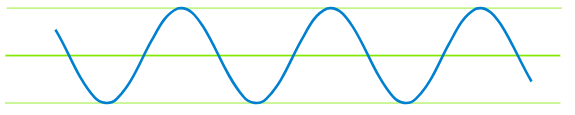What happens when we emit these two signals simultaneously or if we add them together?

When we emit these two signals at the same moment of time, we get a new signal which is the sum of the amplitude of these two signals. This is so because these two signals are being added together.

Sum both the signals: z(t) = x(t) + y(t)If we are given only one signal(which is the sum of signals x(t) and y(t)). Can we recover the original signals x(t) and y(t)?

Yes. That’s what a Fourier transform does. It takes up a signal and decomposes it to the frequencies that made it up.

In our example, a Fourier transform would decompose the signal z(t) into its constituent frequencies like signals x(t) and y(t).

What Fourier transform does is It kind of move us from the time domain to the frequency domain.Credits: eenewsanalog

In case, If anyone is wondering, What if we want to go back from the frequency domain to the time domain?

We can do so by using the Inverse Fourier transform(IFT).

# Maths you need to know.

“Any continuous signal in the time domain can be represented uniquely and unambiguously by an infinite series of sinusoids.”

What does this mean?

It means that, If we have a signal which is generated by some function x(t) then we can come up with another function f(t) such that :So, It doesn’t matter how strong the signal is, we can find a function like f(t) which is a sum of an infinite series of sinusoids that will actually represent the signal perfectly.

Now, the question that arises now is, How do we find the coefficients here in the above equation because these are the values that would determine the shape of the output and thus the signal.So, to get these coefficients we use Fourier transforms and the result from the Fourier transform is a group of coefficients. So, we use X(w) to denote the Fourier coefficients and it is a function of frequency that we get by solving the integral such that :

The Fourier transform is represented as an indefinite integral:

X(w) : Fourier Transform x(t) : Inverse Fourier transformFourier Transform

and

Inverse Fourier transform

Also, when we actually solve the above integral, we get these complex numbers where a and b correspond to the coefficients that we are after.

The continuous Fourier transform converts a time-domain signal of infinite duration into a continuous spectrum composed of an infinite number of sinusoids. In practice, we deal with signals that are discretely sampled, usually at constant intervals, and of finite duration or periodic. For this purpose, the classical Fourier transform algorithm can be expressed as a Discrete Fourier transform (DFT), which converts a finite sequence of equally-spaced samples of a function into a same-length sequence of equally-spaced samples of the discrete-time Fourier transform:So, this is essentially the Discrete Fourier Transform. We can do this computation and it will produce a complex number in the form of a + ib where we have two coefficients for the Fourier series.

Now, we know how to sample signals and how to apply a Discrete Fourier Transform. The last thing we would like to do is, we would like to get rid of the complex number i because it's not supported in mllib or systemML by using something known as Euler's formula which states :So, If we plug Euler’s formula in the Fourier Transform equation and solve it, it will produce a real and imaginary part.As you can see X consists of a complex number of the format a+ib or a-ib. So if you solve the above equation you will get the Fourier coefficients a and b.Now if you just put the values of a and b in the equation of f(t) then you can define a signal in terms of its frequency.

In general practice, we use Fast Fourier Transformation(FFT) algorithm which recursively divides the DFT into smaller DFT’s bringing down the needed computation time drastically. The time complexity of DFT is 2N² while that of FFT is 2NlogN.

# Why are cosine and sine functions used when representing a signal?

While Sine and Cosine functions were originally defined based on right-angle triangles, looking at that point of view in the current scenario isn’t really the best thing. You might have been taught to recognize the Sine function as “opposite by hypotenuse”, but now it’s time to have a slightly different point of view.

Consider the unit circle :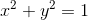on a Cartesian plane. Suppose a line passing through the origin makes an angle θ with the 𝑥-axis in a counterclockwise direction, the point of intersection of the line and the circle is (cos⁡θ, sin⁡θ).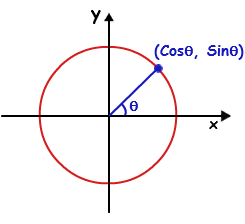Think about it. Does this point of view correlate with the earlier one? Both of the definitions are the same.

Suppose we start to spin the line, by making θ increase linearly. You’d get something like this: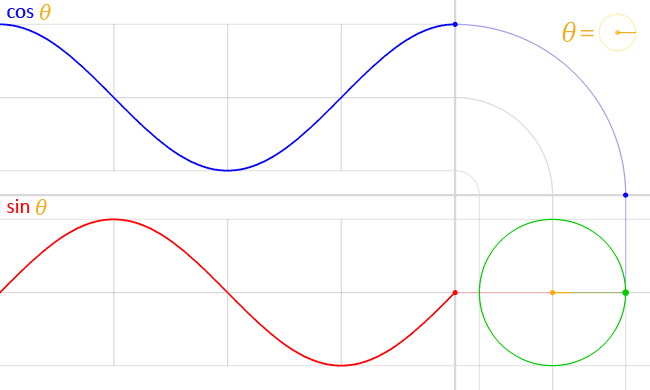Credits: stackexchange

The Sine and Cosine functions are arguably the most important periodic functions in several cases:

1. The periodic functions of how displacement, velocity, and acceleration change with time in SHM oscillators are sinusoidal functions.

2. Every particle has a wave nature and vice versa. This is de Broglie's Wave-Particle duality. Waves are always sinusoidal functions of some physical quantity (such as Electric Field for EM Waves, and Pressure for Sound Waves).

The sound itself is a pressure disturbance that propagates through material media capable of compressing and expanding. It’s the pressure at a point along with the sound wave that varies sinusoidally with time.

# Convergence in Fourier transformation

If a point travels around a circle at a constant speed, its height above the ground traces a sine function. The speed at which the point moves corresponds to the frequency and the radius of the circle corresponds to the amplitude.Almost a discrete waveform.

Because of the Fourier theorem, we can generate any signal with circles of appropriate frequencies and radii.

I used Dan Shiffman’s code from coding challenge #125 to make the animations. You can get the javascript code from his GitHub and can try it yourself.

# Fourier Transformation in AI

Fourier Transformation is a linear function, to induces non-linearity. Convolutions are used.

Fourier Transformation of the product of 2 signals is the convolution of the 2 signals.

Let x(t) and y(t) be two functions with convolution X(t)*Y(t), and F represents Fourier transformation, then

F{x(t).y(t)} = X(t)*Y(t)

Remember that a convolution in the time domain is a multiplication in the frequency domain. This is how Fourier Transform is mainly used in machine learning and more specifically deep learning algorithms.

I’ll take Convolutional Neural Networks, CNNs as an example;

90% of computations in CNNs are convolutions and there have been many approaches to reduce the intensity of such computations, one of them is Fast Fourier Transform (FFT).

Instead of convolutions, the input and filter matrices are converted into the frequency domain by FFT, to do multiplications. Then, the output is converted back into the time domain by Inverse FFT (IFFT).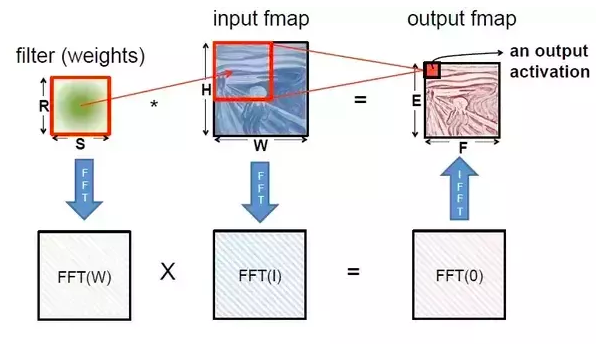Another use of FFT is that it can be used for dimensionality reduction or feature extraction.

When each sample in the dataset is a signal (time series, images, etc.), it may consist of thousands of samples. But they might actually correspond to just a few points in the Fourier domain (especially if there is some periodicity). This simplifies the problem a lot.

Or sometimes using the Fourier domain might provide translation-invariance. That is, even if there are lags between the signals, such variances will not affect their presentation in the Fourier domain.

# Python implementation of Fourier Transform

The simplest possible implementation of FFT can be done using NumPy and scipy python libraries.

```%matplotlib inline
import numpy as np
import matplotlib.pyplot as plt
import scipy.fftpack

# Number of samplepoints
N = 600
# sample spacing
T = 1.0 / 800.0
x = np.linspace(0.0, N*T, N)
y = np.sin(50.0 * 2.0*np.pi*x) + 0.5*np.sin(80.0 * 2.0*np.pi*x)
yf = scipy.fftpack.fft(y)
xf = np.linspace(0.0, 1.0/(2.0*T), N/2)

fig, ax = plt.subplots()
ax.plot(xf, 2.0/N * np.abs(yf[:N//2]))
plt.show()```FFT plot

# Conclusion

The FFT is used in digital recording, sampling, additive synthesis, and pitch correction software.

The FFT’s importance derives from the fact that it has made working in the frequency domain equally computationally feasible as working in the temporal or spatial domain. Some of the important applications of the FFT include:

• Fast large-integer and polynomial multiplication

• Efficient matrix-vector multiplication for Toeplitz, circulant, and other structured matrices

• Filtering algorithms

• Fast algorithms for discrete cosine or sine transform (e.g. Fast Discrete Cosine Transform used for JPEG and MPEG/MP3 encoding and decoding).

• Fast Chebyshev approximation.

• Solving difference equations.

• Computation of isotopic distributions.

Well, that’s all for this article hope you guys have enjoyed reading it and I’ll be glad if the article is of any help. Feel free to share your comments/thoughts/feedback in the comment section.